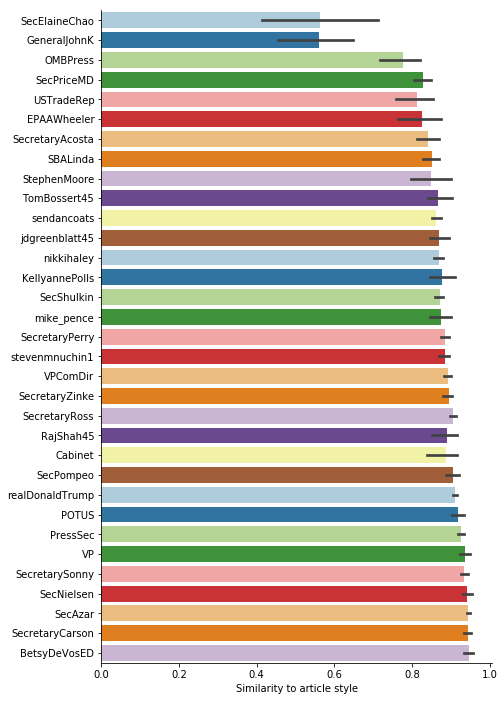David Robinson did a nice writeup of using his R package to analyze who wrote the “I Am Part of the Resistance Inside the Trump Administration” op-ed in NYTimes. His approach was with TF-IDF of the words.

I wanted to try this with different text statsistics of the linguistic features instead, since I’m guessing word usage will not give the author away. And in Python of course.

import spacy
import re

import pandas as pd
import numpy as np
from textstat.textstat import textstat
from sklearn.metrics.pairwise import cosine_similarity
import seaborn as sns
import matplotlib.pyplot as plt
plt.rcParams['figure.dpi'] = 180

from spacy.attrs import ORTH

pd.options.display.max_columns = 999
pd.options.display.max_rows = 999



Here’s a function that takes a arbritary text, and calculates various text statistics. Stuff like mean sentence lenght, variance in sentence lenght, different text difficulty measures from the textstat package. And of course the usage of how much different part of speech is present through the package Spacy.

def linguistic_features(text):

""" Takes a text as input and returns various linguistic features of it """

doc = nlp(text)

# Some standard statistics
sent_lens = [len(s) for s in doc.sents]
mean_sentence_length = np.mean(sent_lens)
sentence_std = np.std(sent_lens)
counts = doc.count_by(ORTH)
n_sentences = len(list(doc.sents))

def per_sentence(char):
""" Count occurances and divide by number of sentences """
try:
return counts[doc.vocab.strings[char]] / n_sentences
except KeyError:
return 0.0

all_caps_count = 0
pos_counts = {}
for token in doc:
# Count capitalized words
if token.text.isupper():
all_caps_count += 1
# Collect POS statistics
try:
pos_counts[token.pos_] += 1
except KeyError:
pos_counts[token.pos_] = 1
pos_per_sent = {f'{k}_per_sent': v / n_sentences for k, v in pos_counts.items()}

return {
'smog_index': textstat.smog_index(text),
'coleman_liau_index': textstat.coleman_liau_index(text),
'difficult_words_per_sent': textstat.difficult_words(text) / n_sentences,
'linsear_write_formula': textstat.linsear_write_formula(text),
'dots_per_sent': per_sentence('.'),
'commas_per_sent': per_sentence(','),
'colons_per_sent': per_sentence(':'),
'bindings_per_sent': per_sentence('-'),
'long_bindings_per_sent': per_sentence('–'),
'quotes_per_sent': per_sentence('"'),
'questions_per_sent': per_sentence('?'),
'exclamations_per_sent': per_sentence('!'),
'mean_sentence_length': mean_sentence_length,
'sentence_std': sentence_std,
'uppercase_words_per_sent': all_caps_count / n_sentences,
**pos_per_sent

}


In lack of time to find good text samples of the White House officials I’ve used their Twitter accounts. This is of course a stretch since there’s no guarrantees that the style you use on Twitter would match the style you use when writing an op-ed. But maybe, just maybe it matches enough?

# List of White House officials twitter accounts to examine
wh_officials = [
'TomBossert45',
'jdgreenblatt45',
'VPComDir',
'SecPompeo',
'RajShah45',
'SecAzar',
'SecNielsen',
'SecretarySonny',
'SecretaryRoss',
'OMBPress',
'EPAAWheeler',
'SecShulkin',
'SecretaryPerry',
'SecPriceMD',
'BetsyDeVosED',
'SecretaryCarson',
'SecretaryZinke',
'SecElaineChao',
'POTUS',
'SBALinda',
'SecretaryAcosta',
'Cabinet',
'VP',
'stevenmnuchin1',
'nikkihaley',
'realDonaldTrump',
'mike_pence',
'sendancoats',
'PressSec',
'GeneralJohnK',
'KellyannePolls',
'StephenMoore'
]


We don’t need the urls.

def remove_urls(t):
return re.sub(r'https?:\/\/.*[\r\n]*', '', t, flags=re.MULTILINE)


Let’s take a twitter accounts collected text and chunk it. That way we can see if the similiarity is consistent by boostrapping some sort of confidence of the similarity.

def collect(user):
""" Collect text from twitter account """
dump = ""
for tweet in get_tweets(user, pages=50):
try:
dump += ' ' + remove_urls(tweet['text'])
except:
continue

return dump

def chunk(in_string, num_chunks):
""" Chunk a string onto num_chunks of equal size """
chunk_size = len(in_string) // num_chunks
if len(in_string) % num_chunks: chunk_size += 1
iterator = iter(in_string)
for _ in range(num_chunks):
accumulator = list()
for _ in range(chunk_size):
try: accumulator.append(next(iterator))
except StopIteration: break
yield ''.join(accumulator)


Now we are ready to collect and calculate all the statistics.

data = {}
for o in wh_officials:
print(o)
text = collect(o)
parts = list(chunk(text, 10))

data[o + '_1'] = linguistic_features(parts)
data[o + '_2'] = linguistic_features(parts)
data[o + '_3'] = linguistic_features(parts)
data[o + '_4'] = linguistic_features(parts)

data['article'] = linguistic_features(article_text)

df = pd.DataFrame.from_dict(data, orient='index').fillna(0.0)


There are many similarity measures but here, after min-max normalizing the features – I use cosine distance.

similarities = (
pd.DataFrame(
# Cosine similarity of min-max normalized df
cosine_similarity((df - df.min()) / (df.max() - df.min())),
index=df.index,
columns=df.index
)[['article']]
.query('index != "article"')
)

ax = sns.barplot(
y="index", x="article",
data=(
similarities.reset_index()
.assign(index=lambda r: r['index'].str.split('_').str[0:-1].str.join('_'))
),
order=(
similarities.reset_index()
.assign(index=lambda r: r['index'].str.split('_').str[0:-1].str.join('_'))
.groupby('index').median()
.reset_index()
.sort_values('article')
)['index']
)
ax.set(xlabel='Similarity to article style', ylabel='')
sns.despine()Dropping out of this is that most similar are BetsyDeVosED, SecretaryCarson, SecAzar, SecNielsen, SecretarySonny, and VP Pence. I have no idea if this anywhere near the truth of course. As far as I’ve read, the only name of those that have been speculated is Pence.